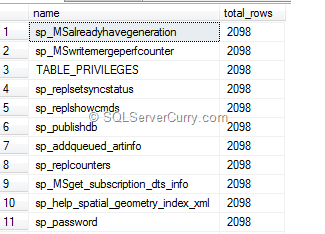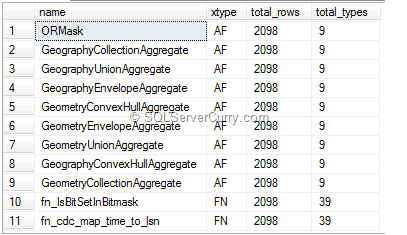### Aggregates without GroupBy SQL Server

When you use aggregate functions along with non-aggregate columns, all non-aggregate columns should be part of GROUP BY Clause. However with the new version of aggregate functions with OVER(), it can be used without the usage of GROUP BY clause.

Suppose you want to return the total number of rows along with a column, you can write count(*) as shown below

select name,count(*) over () as total_rows from sysobjectsThe result shows the value for name column along with total number of rows in total_rows column. Suppose you want to return total number of object types along with a column, you can write count(*) as shown below

select name,xtype,count(*) over () as total_rows,count(*) over (partition by xtype order by xtype) as total_types from sysobjectsThe results shows values for column name , xtype and total number of xtypes for each xtype value. This count differs for each xtype.

This way you can make use of COUNT function without using GROUP BY Clause. From version 2012, you can also make use of SUM function for calculating running total using this technique which you can find at http://www.sqlservercurry.com/2012/08/sql-server-2012-running-total-with-sum.html